# Kotlin print(), println(), readLine(), Scanner, REPL

Published on August 3, 2022By Anupam Chugh
Developer and author at DigitalOcean.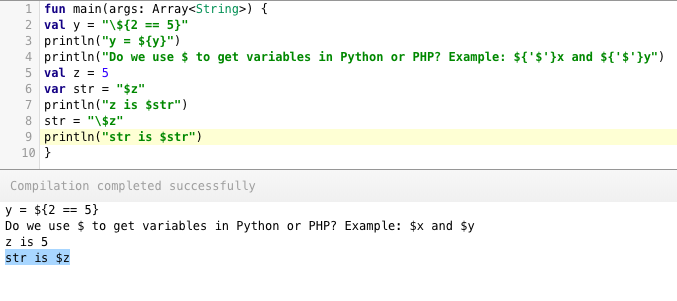While we believe that this content benefits our community, we have not yet thoroughly reviewed it. If you have any suggestions for improvements, please let us know by clicking the “report an issue“ button at the bottom of the tutorial.

Today we will learn how to use Kotlin print functions and how to get and parse user input from console. Furthermore, we’ll look into Kotlin REPL.

## Kotlin Print Functions

To output something on the screen the following two methods are used:

• print()
• println()

The `print` statement prints everything inside it onto the screen. The `println` statement appends a newline at the end of the output. The print statements internally call `System.out.print`. The following code shows print statements in action:

``````fun main(args: Array<String>) {
var x = 5
print(x++)
println("Hello World")
print("Do dinasours still exist?\n")
print(false)
print("\nx is \$x.")
println(" x Got Updated!!")
print("Is x equal to 6?: \${x == 6}\n")
}
``````

To print a variable inside the print statement, we need to use the dollar symbol(\$) followed by the var/val name inside a double quoted string literal. To print the result of an expression we use `\${ //expression goes here }`. The output when the above code is run on the Kotlin Online Compiler is given below.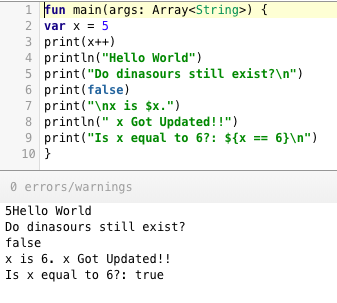### Escape literals and expressions

To escape the dollar symbol and let’s say treat \${expression} as a string only rather than calculating it, we can escape it.

``````fun main(args: Array<String>) {
val y = "\\${2 == 5}"
println("y = \${y}")
println("Do we use \$ to get variables in Python or PHP? Example: \${'\$'}x and \${'\$'}y")
val z = 5
var str = "\$z"
println("z is \$str")
str = "\\$z"
println("str is \$str")
}
``````Note a simple `\$` without any expression/variable set against it implicitly escapes it and treats it as a part of the string only.

### Printing function values

``````fun sumOfTwo(a: Int, b: Int) : Int{
return a + b
}

fun main(args: Array<String>) {
val a = 2
val b = 3
println("Value of \${'\$'}a and \${'\$'}b is : \${sumOfTwo(a,b)}")
println(println("Printing Value of \${'\$'}a and \${'\$'}b is : \${sumOfTwo(a,b)}"))
}
``````

The following output is printed: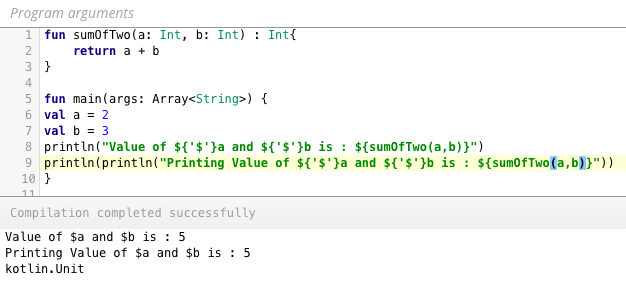Note: Passing a print inside another behaves like recursion. The innermost is printed first. print statement returns a `Unit` (equivalent of void in Java).

## Kotlin User Input

To get the user input, the following two methods can be used:

Note: User input requires a command line tool. You can either use REPL or IntelliJ. Let’s use IntelliJ here.

`readLine()` returns the value of the type String? in order to deal with null values that can happen when you read the end of file etc. The following code shows an example using `readLine()`

``````fun main(args: Array<String>) {
print("Length is \${name?.length}")
}
``````

As you can see we need to unwrap the nullable type to use the String type functions on the property. Use a !! to force convert the String? to String, only when you’re absolutely sure that the value won’t be a null. Else it’ll crash. Converting the input to an Integer To convert the input String to an Int we do the following:

``````fun main(args: Array<String>) {
try {
println("Number multiply by 5 is \${number?.toInt()?.times(5)}")
} catch (ex: NumberFormatException) {
println("Number not valid")
}
}
``````

Again we use the `?.` operator to convert the nullable type to first an Int using `toInt()`. Then we multiply it by 5. Reading Input continuously We can use the do while loop to read the input continuously as shown below.

``````    do {

if (line == "quit") {
println("Closing Program")
break
}

println("Echo \$line")

} while (true)
}
``````

The output of the above in the IntelliJ Command Line is given below.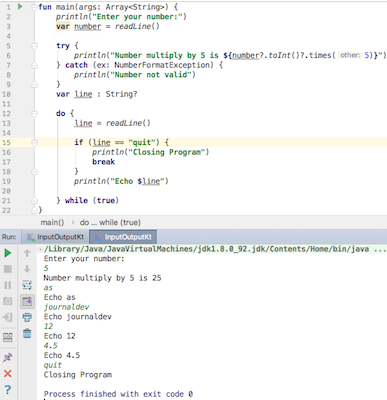### Reading Multiple Values using split operator

We can read multiple values separated by delimiters and save them in a tuple form as shown below.

``````fun readIntegers(separator: Char = ',')

fun main(args: Array<String>) {
try {
val (a, b, c) = readLine()!!.split(' ')
println("Values are \$a \$b and \$c")
} catch (ex: IndexOutOfBoundsException) {
println("Invalid. Missing values")
}

try {
val (x, y, z) = readIntegers()
println("x is \$x y is \$y z is \$z")
} catch (ex: IndexOutOfBoundsException) {
println("Invalid. Missing values")
}
catch (ex: NumberFormatException) {
println("Number not valid")
}

}
``````

The `split` function takes in the character that’ll be the delimiter. `readIntegers()` function uses a map on a split to convert each value to an Int. If you enter values lesser than the specified in the tuple, you’ll get an IndexOutOfBoundsException. We’ve used try-catch in both the inputs. The output looks like this: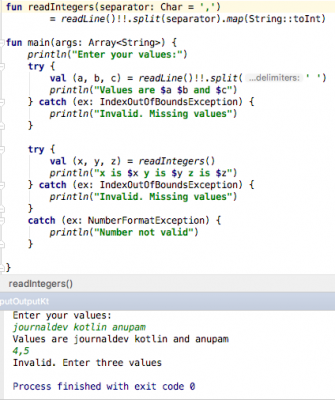Alternatively, instead of tuples, we can use a list too as shown below.

``````val ints: List<String>? = readLine()?.split("|".toRegex())
println(ints)
``````

### Kotlin Scanner Class

To take inputs we can use `Scanner(System.`in`)` which takes inputs from the standard input keyboard. The following code demonstrates the same:

``````fun main(args: Array<String>) {
print("Enter a number: ")

println("You entered: \$integer")
``````

`reader.nextInt()` reads the next integer. `reader.next()` reads the next String. reader.nextFloat() reads the next float and so on. `reader.nextLine()` passes the Scanner to the nextLine and also clears the buffer. The following code demonstrates reading different types of inputs inside a print statement directly.

``````import java.util.*

fun main(args: Array<String>) {

print("Enter a number: ")

try {
println("You entered: \$integer")
} catch (ex: InputMismatchException) {
println("Enter valid number")
}
//move scanner to next line else the buffered input would be read for the next here only.
}

println("Enter a float/boolean :")
try {
} catch (ex: InputMismatchException) {
println("First value should be a float, second should be a boolean. (Separated by enter key)")
}
}
``````

InputMismatchException is thrown when the input is of a different type from the one asked. The output is given below.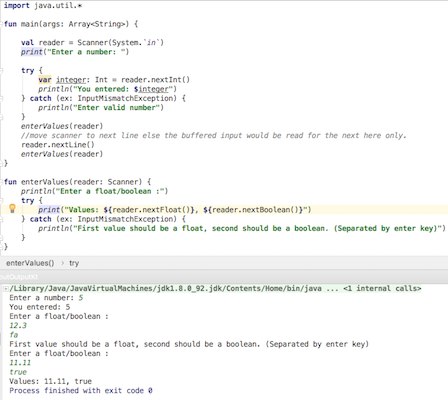## Kotlin REPL

REPL also known as Read-Eval-Print-Loop is used to run a part of code in an interactive shell directly. W e can do so in our terminal/command line by initiating the kotlin compiler.

### Installing the Command Line Compiler

We can install command line compiler on Mac/Windows/Ubuntu as demonstrated here. Typically, on a mac, we can use HomeBrew on our terminal to install the kotlin compiler.

``````brew update
brew install kotlin
``````

Once it is done, start the REPL by entering `kotlinc` in your terminal/cmd Following is my first code in the REPL.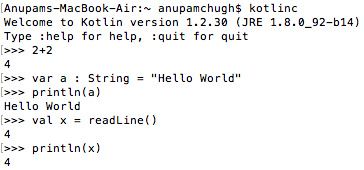That’s all for Kotlin print functions and quick introduction of Kotlin REPL.Developer and author at DigitalOcean.

#### Still looking for an answer?

How to install Android SDK?

- Kirill